Open in App
Not now

# How to delete only one row in CSV with Python?

• Last Updated : 24 Jan, 2021

Prerequisites: pandas

One can open and edit CSV files in Python via Pandas library. While editing the file one might want to remove the entire row in the file. Following are some different approaches to do the same:

Data set in use: iris.csv dataset

### Method 1: Using slicing

This method is only good for removing the first or the last row from the dataset. And the following two lines of code which although means same represent the use of the .iloc[]  method in pandas.

Syntax:

df.iloc[<row_number>, <column_number>]

or

df.iloc[<row_number>]

Approach: To remove first row

• Import the library
• Load the dataset in python
• To remove the first-row using slicing. Since the index column by default is numeric, hence the index label will also be integers.

(0 indexes will be removed as in python indexing starts from 0):

Program:

## Python3

 `import` `pandas as pd`` ` `url ``=` `"https://gist.githubusercontent.com/curran/a08a1080b88344b0c8a7/raw/0e7a9b0a5d22642a06d3d5b9bcbad9890c8ee534/iris.csv"``df ``=` `pd.read_csv(url)`` ` `df ``=` `df.iloc[``1``:]`` ` `print``(df)`

Output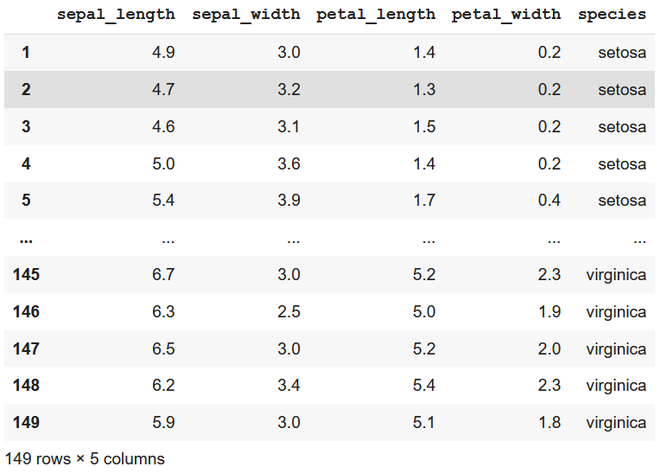removing first row

Approach: To remove the last row

• import the library
• load the dataset in python
• to remove the last-row using slicing. As the index column by default is numeric, hence the index label will also be integers.

(here -1 represents the last row of the data)

Program:

## Python3

 `import` `pandas as pd`` ` `url ``=` `"https://gist.githubusercontent.com/curran/a08a1080b88344b0c8a7/raw/0e7a9b0a5d22642a06d3d5b9bcbad9890c8ee534/iris.csv"``df ``=` `pd.read_csv(url)`` ` `df ``=` `df.iloc[:``-``1``]`` ` `print``(df)`

Output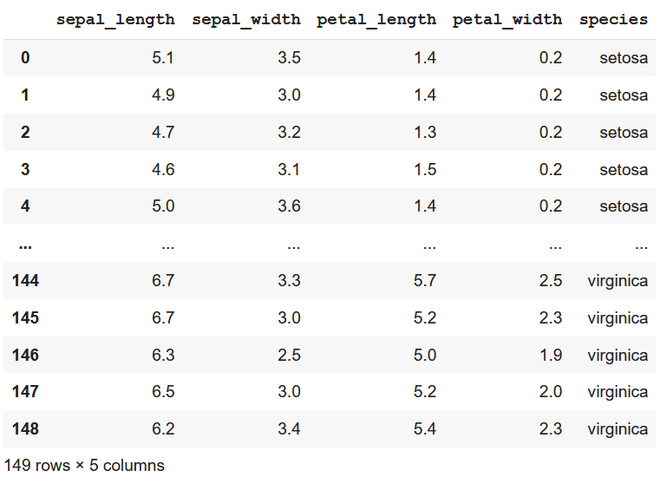removing last row

### Method 2: Using drop() method

Removing using Label means the name of the row is specified in the code whereas using indexing means the index(position/ row number starting from 0) of the row is specified in the code.

Data set in use: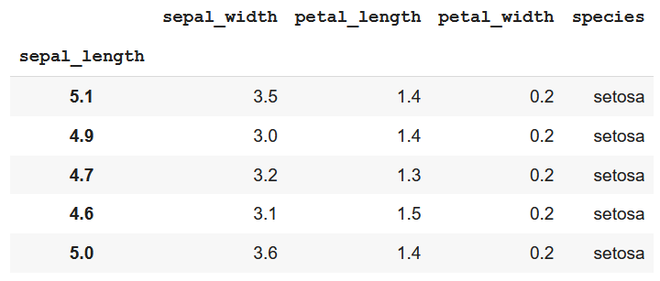subset – top 5 items

Approach: Using row label

• Import pandas library
• Select required data
• With the use of row label (here 5.1) dropping the row corresponding to the same label. Label can be of any data type (string or integer or float etc).

Program:

## Python3

 `import` `pandas as pd`` ` `url ``=` `"https://gist.githubusercontent.com/curran/a08a1080b88344b0c8a7/raw/0e7a9b0a5d22642a06d3d5b9bcbad9890c8ee534/iris.csv"``df ``=` `pd.read_csv(url)`` ` `# 2.``df_s ``=` `df[:``5``]`` ` `# 3.``df_s.set_index(``'sepal_length'``, inplace``=``True``)`` ` `# 4.1.``df_s ``=` `df_s.drop(``5.1``)`` ` `print``(df_s)`

Output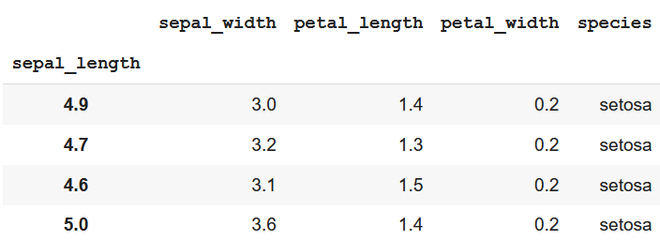using row label

Approach: Using row index

• Import pandas library
• Select required data
• With the use of row index one needs to pass the index of the row to be removed.

df.index[ ] takes index numbers as a parameter starting from 1 and onwards whereas in python indexing starts from 0.

Program:

## Python3

 `import` `pandas as pd`` ` `url ``=` `"https://gist.githubusercontent.com/curran/a08a1080b88344b0c8a7/raw/0e7a9b0a5d22642a06d3d5b9bcbad9890c8ee534/iris.csv"``df ``=` `pd.read_csv(url)`` ` `df_s ``=` `df[:``5``]`` ` `df_s.set_index(``'sepal_length'``, inplace``=``True``)`` ` `df_s ``=` `df_s.drop(df_s.index[``1``])``#df_s.drop(df_s.index,inplace = True)`` ` `print``(df_s)`

Output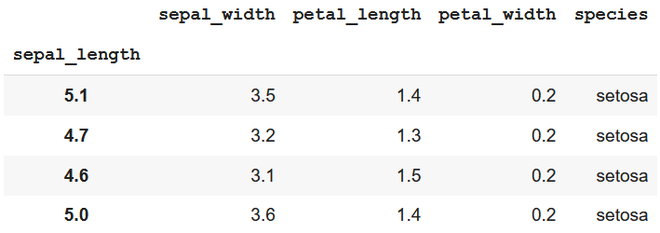using row index

### Method 3: Removing using Conditions

Dataset in use: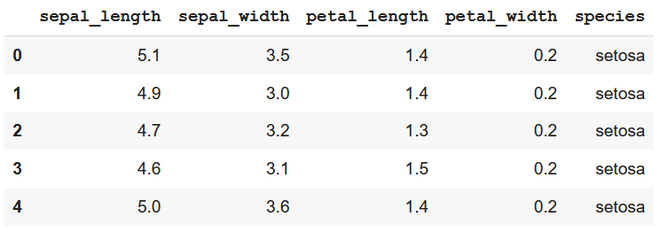subset – top 5 items

Approach 1:

• Import module
• Select required data
• Find the row that specifies the specified condition
• Use drop() method and pass the index of the fetched row as a parameter in the drop method.

Program:

## Python3

 `import` `pandas as pd`` ` `url ``=` `"https://gist.githubusercontent.com/curran/a08a1080b88344b0c8a7/raw/0e7a9b0a5d22642a06d3d5b9bcbad9890c8ee534/iris.csv"``df ``=` `pd.read_csv(url)`` ` ` ` `df_s1 ``=` `df[:``5``]`` ` ` ` `df_s1 ``=` `df_s1.drop(df_s1[(df_s1.sepal_length ``=``=` `4.7``) &``                         ``(df_s1.petal_length ``=``=` `1.3``)].index)`` ` `print``(df_s1)`

Output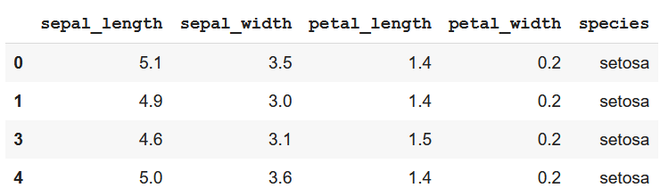first method – conditional removal

Approach 2:

• Import module
• Select required data
• Find the row that specifies the specified condition using query() method
• Use drop() method and pass the index of the fetched row as a parameter in the drop method.

Program:

## Python3

 `import` `pandas as pd`` ` `url ``=` `"https://gist.githubusercontent.com/curran/a08a1080b88344b0c8a7/raw/0e7a9b0a5d22642a06d3d5b9bcbad9890c8ee534/iris.csv"``df ``=` `pd.read_csv(url)`` ` `df_s1 ``=` `df[:``5``]`` ` `df_s1 ``=` `df_s1.drop(df_s1.query(``'sepal_length==5.0'``).index)`` ` `print``(df_s1)`

Output: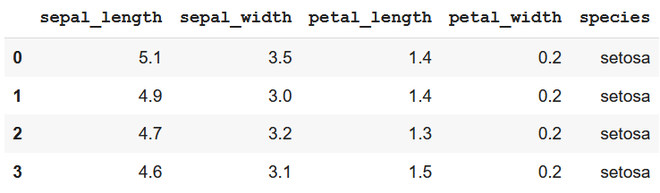second method – conditional removal

My Personal Notes arrow_drop_up